# Forbidden Zone of Roulette Wheels

## Introduction

From thought experiments to movies, the roulette wheel represents pure chance at its finest. There are 38 slots into which the ball could land, and all are equally likely. Thus, a roulette wheel can be treated as a random number generator. From the tense moments in Las Vegas when life savings are on the line, to the cool and calm James Bond who collects his roulette winnings, it seems the outcome is merely determined by luck.

However, roulette is a game governed by classical mechanics, and classical mechanics is at its heart deterministic. If one knows all the relevant information of initial states precisely enough, then one could - in theory - compute the final destination of the ball. Of course, such precise measurements and detailed calculations are impossible even in a laboratory setting, let alone in a casino. The chaotic motion of the ball as it hits the bumpers and scatters across the wheel interfere with any prediction. In spite of this, it is possible to create a prediction that is 'good enough.' By predicting the most likely region of the wheel in which the ball will land, though any single victory is not guaranteed, the odds can be shifted in favor of the player.

There are several methods of arriving at such a prediction. From visual cues to the use of lasers, there are many people online willing to sell such (illegal) systems for a chunk of change. The theory behind these tools is of far more academic value than the tools themselves. The method of prediction we will focus on is using tilt to find a bias in the wheel itself. If the ball is inherently more likely to land in one section of the wheel than another, then the 'pure chance' of the wheel has broken down. As we will see, if the wheel is not perfectly level, such a bias appears. Even with minor tilt, the wheel can create a forbidden zone where the ball is very unlikely to land.

We will begin by analyzing the physics of the roulette wheel, using the work of Thorp  and Eichberger . Though the essence of roulette is simple, in order to make meaningful predictions, friction and air resistance need to be taken into account. These considerations will lead to a forbidden zone: regions in which the ball will not leave the rim of the wheel. After looking at the theory behind the forbidden zone, we will look at an experiment outlined by Dixon  that shows how this forbidden zone translates into biased results.

## Physics of Roulette

### Friction and Drag

At its heart, the physics of an idealized roulette wheel is fairly simple. The basic geometry of the roulette wheel is illustrated in Figure 1. The wheel contains a rim around the outside, along which the ball initially rolls. The wheel itself is tilted inward, as shown in Figure 2, and thus at some point the ball will leave the rim and head towards the center of the wheel. At this point, the ball encounters a set of bumpers, whose purpose is to chaotically scatter the ball. Finally, the ball reaches the innermost part of the wheel, with 38 equally sized slots into which the ball can land.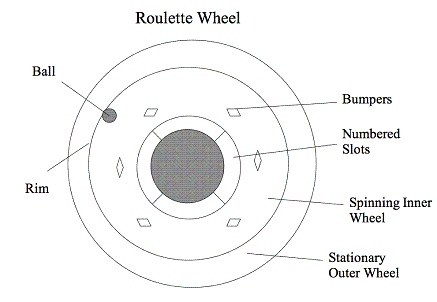Fig. 1: Diagram of Roulette Wheel

Basically, if we ignore friction, drag, and tilt, the wheel itself simply rotates in one direction, and the ball rolls around the rim in the other direction. This motion is determined by the initial angular velocity of the ball and the initial angular velocity of the wheel. With no friction or drag, the ball never loses energy, and never leaves the rim. Thus the idealized roulette wheel is quite oversimplified.

In order to understand how and when the ball leaves the rim of the wheel, we must take friction and drag into account. Using the detailed analysis of Eichberger, the equation of motion for the wheel without tilt is: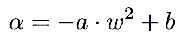Here ω is the angular velocity of the ball, and α is the angular acceleration of the ball. The constants a and b control the strength of the effects due to both friction and drag. How do we know this is the proper description of the roulette wheel? First off, in the case that a=0 and b=0, then α=0, and the angular momentum of the ball is conserved. This is clearly just the frictionless case we mentioned above, so this equation is consistent with our previous analysis.

The acceleration is controlled by a constant term and an ω-squared term. Since we imagine the ball to be rolling quickly around the rim, the air drag is treated as quadratic in velocity. Thus, 'a' contains the drag. The friction can be better understood through the force diagram in Figure 2. The wheel touches both the rim and track, with normal forces against each surface. As the ball rotates around the track, the contact point with the track is treated as not slipping. In that case, as can be verified with a moment's thought, the contact point against the rim must slip. Eichberger analyzes the friction in the non-inertial reference frame of the ball, leading to the centrifugal pseudo-force shown.

Taking both normal forces, we can rewrite them into two terms: one which is constant and the other which is proportional to ω-squared. The steps in this analysis are presented clearly in Eichberger's paper, though we skip the details here. Eichberger's stated result for the constants (for a 'normal' roulette wheel as pictured in Figure 1 under 'normal' atmospheric conditions) is a=.0225 and b=.075 1/s2. Thus the no-tilt equation can be written as: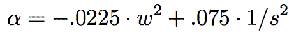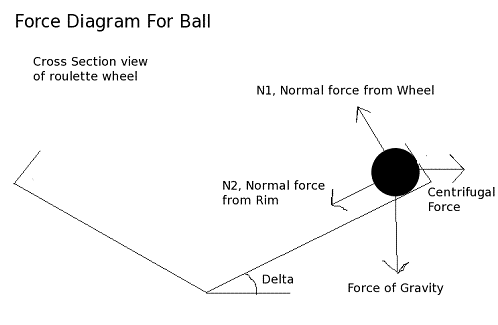Fig. 2: Cross-section of Wheel

### Tilt

The behavior of the roulette wheel becomes far more interesting when it is tilted, especially when our goal is finding bias. To understand this situation, we introduce the coordinate Θ. By tilting the wheel, the symmetry of the situation is broken. Using this broken symmetry, we aid our analysis by defining the angle of the ball, Θ, as the angle of the ball away from the lowest point of the roulette wheel.

The angular acceleration of the ball, α, will now depend not just on the speed of the ball, but also on the location, theta. Why is there a dependence? Well, just as for a ball heading up an incline, the ball will decelerate as it heads from the lower side to the higher side of the roulette wheel. Likewise, as the ball moves from the highest to the lowest point, it will accelerate. The question now becomes how to formulate this dependence.

With some thoughts, the general form of this dependence becomes apparent. The gravitational energy of the ball goes as -cos(Θ). This is just the height dependence of a particle going around a tilted circle, with Θ = 0 at the lowest point. The angular acceleration of the ball goes as the negative derivative of potential energy with respect to angle, which is -sin(Θ). Putting such a term into the roulette equation, we are led to: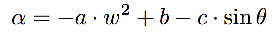With careful analysis of the situation, using geometry and angular kinematics, Eichberger found an equation for c.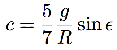Here, R is the radius of the roulette wheel, and ε is the angle of tilt of the wheel. We now have the tools we need to analyze the motion of the roulette ball on a tilted wheel.

### Where the ball leaves the Rim

To predict the outcome and bias of a tilted roulette wheel, it is a necessary intermediate step to know where the ball will leave the rim of the wheel. A bias in the location of the departure point will lead to a bias in the results of the wheel.

Since we know the acceleration of the ball, it is possible to find ω(Θ), the function for the angular velocity of the ball. Leaving out the gritty details of the math - which can be found in Eichberger's paper - this function is found to be: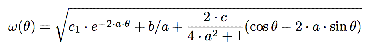where c1 is a constant which depends upon the initial angular velocity and the constants a, b, and c via: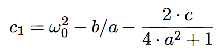We now have an equation for the velocity of the ball. The next step is to find the critical velocity at which the ball will fall from the rim. We can find this using the equation for the normal force against the rim, illustrated in Figure 2. When the normal force N1 goes to zero, the ball will leave the rim. From Eichberger, we find the equation for this normal force.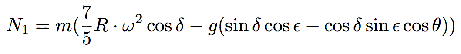For N1=0, the ball will leave the rim of the track, and we label this velocity as wf. It is easy to find the following expression for wf.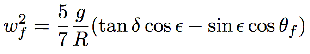This angular velocity will occur at Θf such that ωf = ω(Θf). The easiest way to find Θf is to plot ωf and ω(Θ) and look for where they cross. In fact, the situation can be simplified further by changing the condition from ωf = ω(Θf) to the equivalent equation g1f) = g2f). These new functions are defined to be: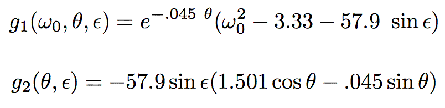These two equations were found from ω and ωf in order to isolate the sinusoidal terms in one of the functions. This will add to the clarity of the graph. These new functions also contain the experimental values of the constants such as a, b, and c, which can be found in Eichberger's paper. To find the crossing points of the two functions, we plot them in Fig. 3. For this graph, we use the reasonable initial angular velocity of 4.5 radians per second. We show the results for two angles of tilt, centering the graph around the first crossing-point.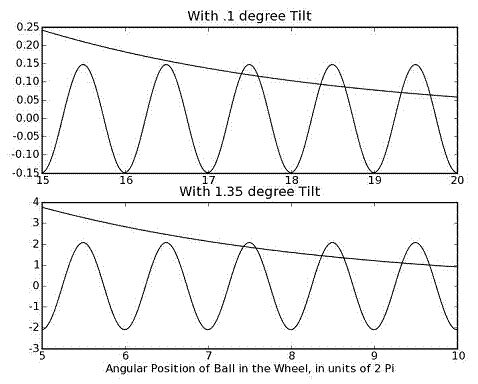Fig. 3: Finding the Departure Angle of the Ball

Looking at the graphs, we find that the initial crossings both occur near the peaks of the sinusoidal wave. Noting that the x-axes are given in units of 2 π, we find that Θf for both cases is near the high point of the wheel. By changing the initial velocity of the system slightly, we can imagine changing the exponential curve. However, wherever we move those curves and however we manipulate the initial conditions, we see the lines can only cross in a relatively narrow section of the wheel. The regions of the roulette wheel just beyond a peak will never be the location for the first intersection. A shadow is effectively created over part of the wheel, in which the ball will never be found to leave the rim. This is known as the forbidden zone in the departure point, and its bias is extreme both for cases of large tilt and small tilt. The motion of the ball after it leaves the rim, as it hits bumpers and scatters, will tend to diminish any bias. However, a strong bias in the departure point of the ball should lead to a remaining bias in the landing location of the ball. We turn to the experimental evidence of such a forbidden zone in the landing location of the ball in the next section.

## Testing the Forbidden Zone

We now have an understanding of how the tilt of a roulette wheel leads to a bias in the departure point of the ball from the rim. However, the motion of the ball as it bounces off the bumpers before landing in a slot is seemingly chaotic. Will this be enough to outweigh the bias of a tilt? Since this clearly depends on the amount the wheel is tilted, perhaps the proper question is: how much tilt is needed to create a noticeable bias? The British National Weights and Measures Laboratory (NWML) performed just such an experiment in 2005 . In this section, we will examine their results.

Table 1: Results of spins on tilted roulette wheels
Section No Tilt .1° Tilt .25° Tilt .5° Tilt 1.35° Tilt
1 23 31 39 34 40
2 18 31 30 48 37
3 24 28 28 21 11
4 22 17 6 9 8
5 22 10 11 16 10
6 28 19 10 7 10
7 18 22 14 13 22
8 21 18 38 28 38
Total Spins 176 176 176 176 176
χ2 3.36 17.8 55.9 62.2 64.1
Expectation for Bias 15% 98% 99.9% 99.9% 99.9%

### The Experiment

For this experiment, a regulation roulette wheel was given a certain tilt (0°, .1°, .25°, .5°, and 1.35°). The wheel was then spun 176 times, and the results were recorded of where the ball landed. For the purposes of recording the results, the wheel was split into eight equal sections - in the stationary reference frame of the table, and the ball was always started in section 5. It is much easier to detect a tilt-based bias by looking at these stationary sections, ignoring the details of predicting how the wheel itself spins and which numbered slots will be where in the final state. The results of a large number of spins were binned and analyzed for statistical bias. The results of this experiment for several amounts of tilt are shown in Table 1 and also plotted in Fig. 4.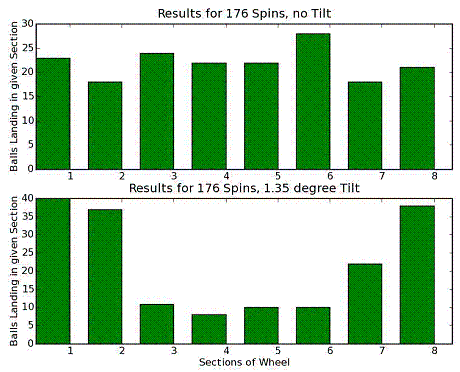Fig. 4: Plot of Results with Tilt and no Tilt

### Analyzing the Results

Looking at the results in the graph, it is easy to qualitatively see bias in the case of extreme tilt. For an ideal, level roulette wheel, it would be expected that each of the eight sections of the wheel would be as likely a landing place as any. We would expect only minor differences in the number of balls landing in each section. In the level case, we see that this is approximately true. However, looking at the case of 1.35° tilt, it becomes apparent that sections 4, 5, and 6 are greatly disfavored, and sections 8, 1, and 2 are greatly favored.

The quantitative bias of the results can be found using chi-square analysis. This is simply a statistical method of determining the chance that results came about through the hypothesized probability distribution (in our case, an even chance for each eight sections). These results are given by Dixon, and they are shown at the bottom of Table 1. As we see, for the level case, the chance of bias was determined to be a mere 15%. However, the chance of bias for the case of 1.35° tilt was greater than 99.9%.

Above, we saw that tilt gave a strong bias in the departure point of the ball from the rim. For the results here of 1.35° tilt, it is clear that the bias remains despite the scattering of the falling ball. Even for quite small angles of tilt, we see that the bias remains. For .1° the chance the final results were due to bias were still overwhelming at 98%.

## Conclusion

We have seen that the tilt of a roulette wheel creates a marked bias in the 'random' results. From the theoretical standpoint, we were able to follow Eichberger's analysis of the equations of motion to show that there is only a relatively small region of the roulette wheel in which the ball will leave the rim. This is a powerful constraint, and depending on the angle, can create sizable bias on the wheel. There are certain places on the wheel where the ball will simply not leave the rim, no matter how many times the experiment is repeated. There is a strictly forbidden zone.

This strong bias is quite interesting, though it in itself is not of practical use in Las Vegas. Rather, the final location of the ball is of far more monetary importance than its departure point from the rim. Looking at the experiment done by NWML, it is clear that the forbidden zone carries over into the statistical results of roulette trials. With regard to the landing place of the ball, there is perhaps not a strictly forbidden zone. The ball can, and is found to, land in any of the eight discrete sections. However, statistically, there is a clear bias, as shown in the results in Table 1. Even for small tilts, the chaotic effects of the bumpers on the falling ball cannot overcome the large forbidden zone in the rim-departure point.

While it is clear that a tilted wheel is biased, it is a far different matter to make a useful prediction. The important result in a casino is not where the ball departs from the rim, or even in which section-with respect to the table-the ball lands. The important prediction is the location on the inner, spinning wheel that the ball lands. Which number or set of numbers should one bet on? This is a much more difficult question, and requires knowing very accurately the initial position of the inner wheel as well as its angular velocity.

There are indeed methods of taking advantage of such a bias in a roulette wheel. There are many websites eager to sell the tools and software needed to win big in a casino. Some fraction of these products even work. However, given the strict regulations against cheating at roulette, it is best to keep these results in the realm of theory rather than practice.

© 2007 Andy Hall. The author grants permission to copy, distribute and display this work in unaltered form, with attribution to the author, for noncommercial purposes only. All other rights, including commercial rights, are reserved to the author.

### References

 J. Eichberger, "Roulette Physics," http://www.roulette.gmxhome.de/ (2004).

 P. Dixon, "Roulette Wheel Testing" Report on Stage 3.1 of NWML/GBGB Project Proposal (2005).

 E. O. Thorp, "Optimal Gambling Systems for Favorable Games," Rev. Int. Stat. Inst. 37, 273 (1969).